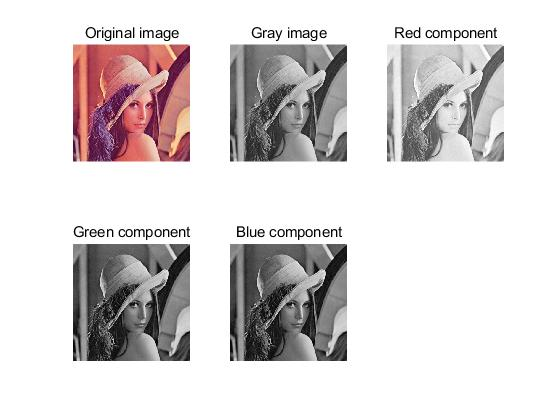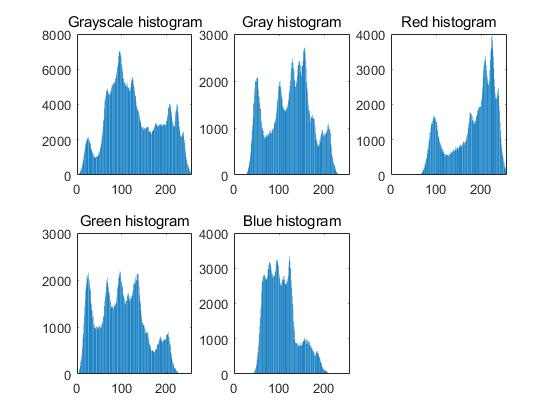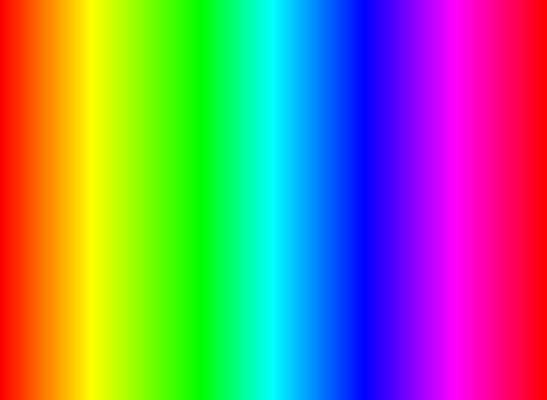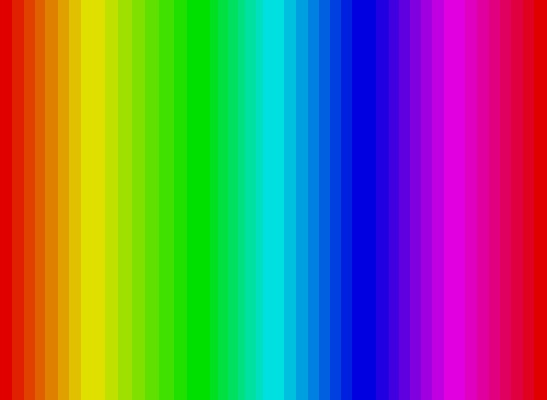• 关于GPU编程的这些资料均是我早期的一些资料，趁出差这段时间整理下，所以就直接复制过来了，其中会有一些...图像的纹理内存的读取方法： 特别注意：gpu上的tex2D（img，x,y）中的x,y坐标对应图像坐标是： X=0~cols，y

关于GPU编程的这些资料均是我早期的一些资料，趁出差这段时间整理下，所以就直接复制过来了，其中会有一些瑕疵，请读者朋友斧正，以下的代码仅仅是验证，在VS上已通过且达到了预期的目的，如果有时间，接下来我会编写并分享使用gpu编程实际应用过程中的经验教训和总结。

图像的纹理内存的读取方法：
特别提示：gpu上的tex2D（img，x,y）中的x,y坐标对应图像坐标是：
X=0~cols，y=0~rows，与img.at<uchar>（x，y）的x,y刚好相反。
一、纹理内存的使用示例：
1、一维纹理内存示例（cu文件，必须先把数据cudaMemcpy到设备上，然后把设备上的该数据绑定到纹理内存）：

texture <unsigned char, 1, cudaReadModeElementType>textImg;
__global__voidCalAngle(int imgH,intimgW, ,unsignedchar*dev_ptr,
)
{
intx = threadIdx.x + blockIdx.x*blockDim.x;
if(x <= (imgH*imgW))
{
dev_ptr[x]= tex1Dfetch(textImg, x);
}
}

void main(Mat  img)
{
imgH=img.rows, imgW=img.cols;
unsignedchar *imgPtr;
imgPtr=img.data;

unsigned char *dataPtr;
cudaMalloc((void**)&dataPtr,imgH*imgW*sizeof(unsigned char));
cudaMemcpy(dataPtr, imgPtr,imgH*imgW*sizeof(unsigned char), cudaMemcpyHostToDevice);
int imageSize = imgH*imgW*sizeof(unsignedchar);
cudaBindTexture(NULL, textImg, imgPtr,imageSize);
unsigned char *dev_ptr;
cudaMalloc((void**)&dev_ptr,imgH*imgW*sizeof(unsigned char));
CalAngle << <imgH,  imgW >> >( imgH, imgW, dev_ptr,);//注意：N，M不能超过某一数值，如1536或者1024，具体多少每种显卡均不一样，有兴趣的读者可以编写简单的语句获得
Mat outMat(imgH,imgW, CV_8UC1);
outMat.setTo(Scalar::all(55));
unsigned char*host_ptr;
host_ptr =outMat.data;
cudaMemcpy(host_ptr,dev_ptr, imgH*imgW*sizeof(unsigned char), cudaMemcpyDeviceToHost);
cudaUnbindTexture(textImg);
cudaFree(dev_ptr);
cudaFree(dataPtr);

Mat testGpu;
absdiff(img,outMat, testGpu);
cout<<"testGPU = "<< testGpu << endl;
}

2、二维纹理内存示例（cu文件，必须先把数据cudaMemcpy到设备上，然后把设备上的该数据绑定到纹理内存）：
以下代码中：针对main函数中的#if1 #else #endif结构中的两种情况，核函数CalAngle中的#if
1 #else #endif结果中#else下的代码可能具有普适性，请自行验证（去掉#if1下的内容及该结构，值保留#else下的内容）。
texture <unsigned char, 2,cudaReadModeElementType> textImg;
__global__voidCalAngle(int imgH,intimgW, ,unsignedchar*dev_ptr,
)
{
intx = threadIdx.x + blockIdx.x*blockDim.x;
if(x <= (imgH*imgW))
{
#if 1  //一维线程块，线程块中的线程是一维线程
int  m = threadIdx.x,   n = blockIdx.x;
//n*imgW + m  (tex2D(textImg, m,n)) 从图像原点按列读取（如imag.at<uchar>（0,0）->(0,1)->(0,2)）
// tex2D(textImg, n,m) 的读取方式是 imag.at<uchar>（0,0）->(1,0)->(2,0)
dev_ptr[x]= tex2D(textImg, m,n);
#else
//二维线程块，线程块中的线程是二维线程(理论上应该也适应上述#if 1“一维线程块，线程块中的线程是一维线程”的情况，请自行验证)
int y = threadIdx.y + blockIdx.y*blockDim.y;
int mn = x + y*blockDim.x*gridDim.x;
if(mn<imgH*imgW)
{
Int  m= mn % imgW,  n = mn / imgW;
dev_ptr[mn]= tex2D(textImg, m, n);
}
#endif
}
}

void main(Matimg)
{
imgH=img.rows, imgW=img.cols;
unsignedchar *imgPtr;
imgPtr=img.data;
unsigned char *dataPtr;
cudaMalloc((void**)&dataPtr,imgH*imgW*sizeof(unsigned char));
cudaMemcpy(dataPtr, imgPtr, imgH*imgW*sizeof(unsignedchar), cudaMemcpyHostToDevice);
int imageSize = imgH*imgW*sizeof(unsignedchar);
cudaBindTexture(NULL, textImg, imgPtr,imageSize);
unsigned char *dev_ptr;
cudaMalloc((void**)&dev_ptr,imgH*imgW*sizeof(unsigned char));
#if 1  //一维线程块，线程块中的线程是一维线程
CalAngle << <imgH,  imgW >> >( imgH, imgW, dev_ptr,);//注意：N，M不能超超过某一数值，如1536或者1024，具体多少每种显卡均不一样，有兴趣的读者可以编写简单的语句获得
#else //二维线程块，线程块中的线程是二维线程
dim3 dev_block((imgH + 31) / 32, 32);
dim3 dev_threads((imgW+31)/32, 32);
CalAngle << < dev_block,  dev_threads >> >( imgH, imgW,dev_ptr,);
#endif
Mat outMat(imgH,imgW, CV_8UC1);
outMat.setTo(Scalar::all(55));
unsigned char*host_ptr;
host_ptr =outMat.data;
cudaMemcpy(host_ptr,dev_ptr, imgH*imgW*sizeof(unsigned char), cudaMemcpyDeviceToHost);
cudaUnbindTexture(textImg);
cudaFree(dev_ptr);
cudaFree(dataPtr);

Mat testGpu;
absdiff(img,outMat, testGpu);
cout<<"testGPU = "<< testGpu << endl;
}

展开全文cuda 深度学习 神经网络 计算机视觉 线程
• ## Matalb--读取图像及像素值

万次阅读 多人点赞 2018-03-22 23:35:11
彩色图像是由G、R、B三中颜色像素合成的，图像矩阵的原点一般算法选择在图像的左上角，向右为x，向下为y，即（0，0）。彩色图像形成的三维数组中前两位表示图像的像素点的坐标，第三位为1时表示是图像中R的，为2时...
    彩色图像是由G、R、B三中颜色像素合成的，图像矩阵的原点一般算法选择在图像的左上角，向右为x，向下为y，即（0，0）。彩色图像形成的三维数组中前两位表示图像的像素点的坐标，第三位为1时表示是图像中R的值，为2时表示G的值，为3时表示B的值。    代码实现如下clc;
clear;
%读入原图像及获取大小
image = imread('lena.jpg');
% 分别读取R、G、B值
image_r=image(:,:,1);
image_g=image(:,:,2);
image_b=image(:,:,3);

image_gray=rgb2gray(image);%将彩图转换为灰度图

hist_image=imhist(image);%计算总图直方图
hist_gray=imhist(image_gray);%计算灰度直方图
hist_r=imhist(image_r);%计算Red部分直方图
hist_g=imhist(image_g);%计算Green部分直方图
hist_b=imhist(image_b);%计算Blue部分直方图
%  测试RGB输出
figure(1);%在窗口1中显示原图、灰度图及R、G、B图
subplot(2,3,1),imshow(image),title('Original image');
subplot(2,3,2),imshow(image_gray),title('Gray image');
subplot(2,3,3),imshow(image_r),title('Red component');
subplot(2,3,4),imshow(image_g),title('Green component');
subplot(2,3,5),imshow(image_g),title('Blue component');
%  画直方图
figure(2);
subplot(2,3,1),bar(hist_image),title('Grayscale histogram');%画总直方图
subplot(2,3,2),bar(hist_gray),title('Gray histogram');%画灰度直方图
subplot(2,3,3),bar(hist_r),title('Red histogram');%画Red部分直方图
subplot(2,3,4),bar(hist_g),title('Green histogram');%画Green部分直方图
subplot(2,3,5),bar(hist_b),title('Blue histogram');%画Blue部分直方图效果如下此图为上图所对应的直方图展开全文• 使用opencv读取视频帧，将该帧保存为jpg后，再用出cv2.imread()读取，打印像素值发现，两个矩阵的元素值是不一样的。但是如果将该帧保存为png，那么再读取像素值是一样的。 这个问题导致我在用jpg图片训练svm后，...
使用opencv读取视频帧，将该帧保存为jpg后，再用出cv2.imread()读取，打印像素值发现，两个矩阵的元素值是不一样的。但是如果将该帧保存为png，那么再读取后像素值是一样的。

这个问题导致我在用jpg图片训练svm后，读取视频帧并裁减了很小一块roi（尺寸12*12）进行的分类结果不对。需要注意下。


展开全文• 一个图像有可能包含数以万计的像素，从根本上说图像就是一系列像素值，所以OpenCV使用数据结构cv::Mat来表示图像矩阵中每一个元素都代表一个像素，对于灰度图像，像素用8位无符号数，0表示黑色，255表示白色。对于...

在计算机视觉应用中，对于图像内容的读取分析是第一步，所以学习高效的处理图像是很有用的。一个图像有可能包含数以万计的像素，从根本上说图像就是一系列像素值，所以OpenCV使用数据结构cv::Mat来表示图像。矩阵中每一个元素都代表一个像素，对于灰度图像，像素用8位无符号数，0表示黑色，255表示白色。对于彩色像素而言，每个像素需要三位这样的8位无符号数来表示，即三个通道（R,G,B），矩阵则依次存储一个像素的三个通道的值，然后再存储下一个像素点。

cv::Mat中，成员变量cols代表图像的宽度（图像的列数），成员变量rows代表图像的高度（图像的行数），step代表以字节为单位的图像的有效宽度，elemSize返回像素的大小，像素的大小 = 颜色大小（字节）*通道数，比如三通道short型矩阵（CV_16SC3）的大小为2*3 = 6，像素的channels方法返回图像的通道数，total函数返回图像的像素数。

闲话少说直接介绍几种读取方式：

RGB图像的颜色数目是256*256*256，本文对图像进行量化，缩减颜色数目到256的1/8（即32*32*32）为目标，分别利用一下几种方法实现，比较几种方法的安全和效率。

1.ptr遍历图像

cv::Mat中提供ptr函数访问任意一行像素的首地址，特别方便图像的一行一行的横向访问，如果需要一列一列的纵向访问图像，就稍微麻烦一点。但是ptr访问效率比较高，程序也比较安全，有越界判断。

[cpp] view
plain copy

int nl= image.rows; //行数
int nc= image.cols * image.channels(); // 每行的元素个数，每行的像素数*颜色通道数（RGB = 3）

for (int j=0; j<nl; j++) {
uchar* data= image.ptr<uchar>(j);
for (int i=0; i<nc; i++) {
// process each pixel ---------------------
data[i]= data[i]/div*div + div/2;
// end of pixel processing ----------------
} // end of line
}
也可以使用：

[cpp] view
plain copy

for (int j=0; j<nl; j++) {
uchar* data= image.ptr<uchar>(j);
for (int i=0; i<nc; i++) {
// process each pixel ---------------------
*data++= *data/div*div + div/2;
// end of pixel processing ----------------
} // end of line
}

2.使用迭代器遍历图像

cv::Mat 同样有标准模板库（STL），可以使用迭代器访问数据。

用迭代器来遍历图像像素，可简化过程降低出错的机会，比较安全，不过效率较低；如果想避免修改输入图像实例cv::Mat，可采用const_iterator。iterator有两种调用方法，cv::MatIterator_<cv::Vec3b> it;cv::Mat_<cv::Vec3b>::iterator it;中间cv::Vec3b是因为图像是彩色图像，3通道，cv::Vec3b可以代表一个像素。

[cpp] view
plain copy

// get iterators
cv::Mat_<cv::Vec3b>::iterator it= image.begin<cv::Vec3b>();
cv::Mat_<cv::Vec3b>::iterator itend= image.end<cv::Vec3b>();

for ( ; it!= itend; ++it) {

// process each pixel ---------------------

(*it)= (*it)/div*div + div/2;
(*it)= (*it)/div*div + div/2;
(*it)= (*it)/div*div + div/2;

// end of pixel processing ----------------
}

3.at方法遍历

cv::Mat也是向量，可以使at方法取值，使用调用方法image.at<cv::Vec3b>(j,i)，at方法方便，直接给i，j赋值就可以随意访问图像中任何一个像素，其中j表示第j行，i表示该行第i个像素。但是at方法效率是这3中访问方法中最慢的一个，所以如果遍历图像或者访问像素比较多时，建议不要使用这个方法，毕竟程序的效率还是比程序的可读性要重要的。下面是完整的调用方法，其运行时间在下面会介绍。

[cpp] view
plain copy

int nl= image.rows; // number of lines
int nc= image.cols; // number of columns

for (int j=0; j<nl; j++) {
for (int i=0; i<nc; i++) {

// process each pixel ---------------------

image.at<cv::Vec3b>(j,i)=    image.at<cv::Vec3b>(j,i)/div*div + div/2;
image.at<cv::Vec3b>(j,i)=    image.at<cv::Vec3b>(j,i)/div*div + div/2;
image.at<cv::Vec3b>(j,i)=    image.at<cv::Vec3b>(j,i)/div*div + div/2;

// end of pixel processing ----------------

} // end of line
}

[cpp] view
plain copy

4.row（i），col(i)

cv::Mat提供image.row(i)，和image.col(j)对图像整行和整列进行处理，处理比较方便，因为很少遇到，所以就没有进行效率比较

程序代码如下（三通道）：

[cpp] view
plain copy

result.row(0).setTo(cv::Scalar(0,0,0));//将第一行数据设为零
result.row(result.rows-1).setTo(cv::Scalar(0,0,0));//将最后一行数据设置为零
result.col(0).setTo(cv::Scalar(0,0,0));//将第一列数据设为零
result.col(result.cols-1).setTo(cv::Scalar(0,0,0));//将最后一列数据设为零

5.高效率图像遍历循环
对于迭代的for循环，外面一层的循环次数越少速度越快，同样是cols*rows*channels()次循环，使用nc = cols，nl = rows*channels()，与使用nc = cols*rows*channels，nl = 1，以及nc = cols，nl =
rows，for函数最里层运行三次颜色的处理。这三种方法最快的是第二种，第三种其次，最慢的是第一种.

[cpp] view
plain copy

int nl= image.rows; // number of lines
int nc= image.cols * image.channels(); // total number of elements per line

if (image.isContinuous())  {
// then no padded pixels
nc= nc*nl;
nl= 1;  // it is now a 1D array
}

int n= static_cast<int>(log(static_cast<double>(div))/log(2.0));
// mask used to round the pixel value
uchar mask= 0xFF<<n; // e.g. for div=16, mask= 0xF0

for (int j=0; j<nl; j++) {

uchar* data= image.ptr<uchar>(j);

for (int i=0; i<nc; i++) {

// process each pixel ---------------------

*data++= *data&mask + div/2;

// end of pixel processing ----------------

} // end of line
}

6.位运算代替乘法和除法

[cpp] view
plain copy

int nl= image.rows; // number of lines
int nc= image.cols * image.channels(); // total number of elements per line
int n= static_cast<int>(log(static_cast<double>(div))/log(2.0));
// mask used to round the pixel value
uchar mask= 0xFF<<n; // e.g. for div=16, mask= 0xF0

for (int j=0; j<nl; j++) {

uchar* data= image.ptr<uchar>(j);

for (int i=0; i<nc; i++) {

// process each pixel ---------------------

*data++= *data&mask + div/2;

// end of pixel processing ----------------

} // end of line
}

运行时间比较，以测试图片为例，（Debug模式下的时间）
ptr函数的两种方法的时间：                using .ptr and [] =3.50202ms                using .ptr and * ++ =3.26124ms   迭代器方法：              using Mat_ iterator =143.06ms   at方法的运行时间：             using at =252.779ms   高效率迭代方法:            using .ptr and * ++ and bitwise (continuous) =2.68335ms   位运算方法：
using .ptr and * ++ and bitwise =2.59823ms

还有一些比较的函数方法，现在只给出运行时间：         using .ptr and * ++ and modulo =3.78029ms         using .ptr and * ++ and bitwise =2.59823ms         using direct pointer arithmetic =2.57317ms         using .ptr and * ++ and bitwise with image.cols * image.channels() =22.864ms         using Mat_ iterator and bitwise =139.92ms         using MatIterator_ =185.996ms        using .ptr and * ++ and bitwise (continuous+channels) =2.11271ms         using input/output images =2.97717ms         using overloaded operators =2.7237ms

源图像：量化处理结果 image1：量化处理后的结果 image2：展开全文opencv
• 很多时候，我们需要读取某个像素值，或者设置某个像素值；在更多的时候，我们需要对整个图像里的所有像素进行遍历。 OpenCV 提供了多种方法来实现图像的遍历。 1 at()函数 函数 at()来实现读去矩阵中的某个像素，...opencv
• 利用opencv读取图像，并转换成二维矩阵的形式，以方便运算处理,返回类型为标准的二维数组，通过行列索引即可访问对应的像素值
• 用zeros（x，y）建立了一个矩阵，把里面对应一些位置改为1，这样就有了下图中上的结果，各个像素值都很正常，imshow出来也没有问题标准的二值图像。然后我就imwrite保存了。结果想继续处理的时候，用imread读取保存...
• ## opencv读取图像的灰度值并显示出来

万次阅读 多人点赞 2018-11-19 10:17:57
通过双层循环，遍历所有的像素值，再输出灰度值即可。图片太大，所有只选择20行和20列进行输出。  昨天要用到图片的灰度值，脑子突然短路了，忘了存储灰度图的数组，存放的就是图片的灰度值，还以为要用什么函数...opencv
• 很多时候， 我们需要读取某个像素值， 或者设置某个像素值； 在更多的时候，我们需要对整个图像里的所有像素进行遍历。OpenCV 提供了多种方法来实现图像的遍历。 1、at()函数 函数 at()来实现读取矩阵中的某个像素...
• 在C++中，读入一幅图像使用语句如下，读入的图像像素为0-...其中图像每个像素的RGB是用3元素向量来存储（B,G,R）的，某一像素点的RGB值读取方式如下： Vec3b& mp = disp_image.at<Vec3b>(j, i); //C+...
• pytorch学习—图像的加载/读取方式(转) 使用pytorch制作图像数据集时，需要将存储在磁盘、硬盘的图像读取到内存中，涉及到图像I/O问题。 在python中，图像处理主要采用的库：skimage, ...像素值范围 图像矩阵表示 ...
• 我们需要读取某个像素值，或者设置某个像素值；在更多的时候，我们需要对整个图像里的所有像素进行遍历。OpenCV 提供了多种方法来实现图像的遍历。 at()函数 函数at（）用于读取矩阵中的某个像素，或者对某个像素...
• 在计算机里，一张尺寸为MxN的图像，可以用一个MxN的矩阵来表示，矩阵元素的表示这个位置上像素的亮度，越大，说明这个点越亮。 新版本的opencv里有了Mat类，Mat是一个非常优秀的图像类，也是一个通用的矩阵...
• 计算机视觉中的图像只是简简单单的多维矩阵，而SimpleITK中的图像是表示一种真实的物理实体，图像中的每一个像素都表示了是真实物理空间中的一个点，不仅仅有像素值，还有着坐标，间距，方向等概念。 Size：图像在各...
• 这个三维数组有三个面,依次对应于红(Red)、绿(Green)、蓝(Blue)三种颜色,而面中的数据则分别是这三种颜色的强度,面中的元素对应于图像中的像素点。设所得矩阵为X三维矩阵(256,256,3) ,X(:,:,1)代表红颜色matlab 工具 存储
• 图像加载问题 使用pytorch制作图像数据集时，需要将存储在磁盘、硬盘的图像读取到内存中，涉及到图像I/O问题。... 像素值范围 图像矩阵表示 skimage io.imread(xxx) numpy.ndarray RGB [0,
•  对于24位的RGB图像，用imread读取的数据为（h，w，3）的三维矩阵，（i， j，1:3）是第i行第j列像素的R、G、B；  用reshape转换为（h×w，3）的二维矩阵后，每一行是一个像素的RGB像素的排列是先列后行，...
• 函数at()对矩阵中某个像素进行读取或者对某个像素进行复制操作。但是效率并不高。 例如：uchar value = grayim.at(i,j); //读出第i行第j列像素值  grayim.at(i,j) = 128; //将第i行第j列像素值设置为128 //...opencv at
• 在对图像像素值进行加减操作时，要注意数据类型，不然就容易造成数据的溢出，计算出的结果并不是你想得到的值，下面给出例子。 首先定义一个矩阵保存为图片： from skimage.io import imread,imsave import ...数据类型 溢出
• * 读取一张灰度图像 read_image (Image, 'fabrik') * 获取图像大小 get_image_size(Image, Width, Height) * 获取区域里每个像素的坐标 * 参数2,3：输出参数 get_region_points(Image, Rows, Columns) * 获取图像...机器视觉 工业视觉
• 使用cv2.imread读取图片将其储存为一个BGR像素值矩阵。 接下来我们看看相关的代码操作： import cv2 # 导入OpenCV库 import numpy as np img = cv2.imread('xxx.jpg', 0) # 读取图片：灰度模式 img = cv2.imread...opencv 计算机视觉 人工智能 深度学习 机器学习
• 用MATLAB获取了一幅彩色图像像素值，得到一个400*300*3的三维矩阵，并保存到txt文档中，怎么写一个c++小程序，把txt中的数据写入到一个三维矩阵中？ %读取第k个图像，所有像素 srcImg = imread(fullfile(SRC, ...c++
• 减少参数以获得仅具有有效像素值的最大矩形图像对并以读取图像的方式排序（第一行的第一个像素，然后是第二行的像素等）。 双线性参数放置在矩阵中。 已完成失真图像的校正通过简单的矩阵乘法和加法。 这导致更快的...matlab
•  在我看来，图像处理基本基于对像素值的处理，那么我们尽可能的用数组来存储像素值，对于OpenCV来说不便于按照我们的做法来对图片进行处理。但是OpenCV给我们集成了cv::Mat::at这样一个结构，用于读取图...OpenCV
• 我最近在做嵌入式图像处理，我的任务是：首先要把一幅图像读入matlab，获取每个像素点的灰度，然后分别在TXT文件中以二进制、十进制和十六进制的数值显示出来。 在matlab中使用imread函数读入一幅图像，得到由...
• 图像加载问题 使用pytorch制作图像数据集时，需要将存储在磁盘、硬盘的图像读取到内存中，涉及到图像I/O问题。 在python中，图像处理主要采用的库：skimage, opencv-python, ... 像素值范围 图像矩阵表示 ...
• ##像素的读取读取第10行第10列的像素值，原点在左上角 img=cv2.imread('imgs/04_img.jpg',1) ##img是矩阵,img[a,b]可以取出第a列第b行的数据， ##读取的值由元组组成，OpenCV对应的是bgr px= img[10,10] print(px)...OpenCV
• 读取像素值时，不同的图像，读取过程有些差别。图像主要是两种：灰度图与RGB图，即黑白图像与彩色图像。 1.黑白图像 注意：y 是行，x 是列，所以先写 y ，再写 x 。 Scalar intensity = image.at<uchopencv 计算机视觉
• ()，下面我们就介绍OpenCV中这两种常用的对指定位置像素值读取和修改操作。 一、at操作 at操作是一种直接简单的对单个像素的操作方式，用于获取图像矩阵某点的值或改变某点的值 对于灰度图读取和修改操作如下：......